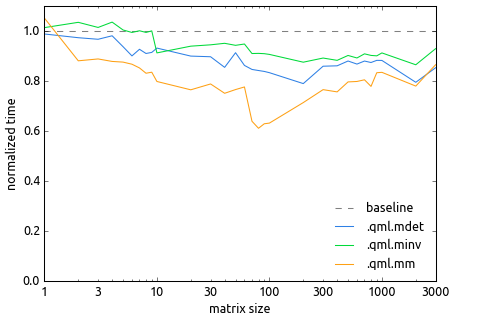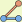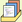# Q Math Library

## Introduction

qml is a library for statistics, linear algebra, and optimization in kdb+. It provides an interface between the q programming language and numerical libraries such as LAPACK.

The current version of the library is 0.7.3 (track updates).

## News

2016-11-22

The main function of the LAPACK wrappers in qml is to convert matrices between q format (list of row vectors) and Fortran format (column-major array). This conversion can be viewed as a combination of a memory copy and a matrix transpose, which is slower than just a linear memory copy and can be tricky to optimize. A typical optimization is to use a blocked algorithm (4 nested loops instead of 2), but the optimal block size depends on the system architecture and the algorithm introduces extra complexity and corner cases.

This conversion doesn't account for most of the runtime of qml functions – the actual LAPACK operation does. For example, in .qml.mm the transpose is O(n²) while the multiplication is O(n³). But it does add substantial overhead.

However, it turns out that in many cases the transpose is unnecessary. We still need to convert between q format (non-contiguous vectors) and Fortran format (contiguous array), but we can do just a memory copy without a transpose and fold the transpose into the actual LAPACK call:

• in .qml.mdet transpose doesn't matter as |A|=|Aᵀ|

• in .qml.minv the input and output transpose can be omitted together as A⁻¹=((Aᵀ)⁻¹)ᵀ

• in .qml.mm we can omit the input and output transpose if we swap the arguments, as AB=(BᵀAᵀ)ᵀ

Here's a graph showing the effectiveness of this optimization (ratio of qml 0.7 run time to qml 0.6 run time) at different matrix sizes when linked against OpenBLAS:Matrix multiplication is the fastest of these operations and involves the largest number of matrices (2 inputs and 1 output), and OpenBLAS is faster than Netlib BLAS, so reducing the overhead makes the most difference to .qml.mm using OpenBLAS: it's 10-60% faster in qml 0.7.

qml is free software with a BSD-style license. It is provided in the hope that it will be useful, but with absolutely no warranty. See the file LICENSE.txt for details.

qml is linked against several other libraries. The copyrights and licenses for these libraries are also listed in LICENSE.txt.

 Current version:Source code on GitHubBinaries on GitHubqml-0.7.3.zip (173.11 KB; 2017-07-25; source code only)qml-0.7.3-bin.zip (3.08 MB; 2017-07-26; binary for kdb+ 3 on w32) Older versions:qml-0.6.zip (143.77 KB; 2015-12-27; source code only)qml-0.5.5.zip (137.99 KB; 2015-06-21; source code only)qml-0.4.zip (99.65 KB; 2013-05-12; source code only) Legacy versions:Source code on GitHubqml-0.3.10.zip (76.88 KB; 2011-07-13; source code only)qml-0.3.8.zip (74.28 KB; 2011-07-08; source code only)qml-0.2.1.zip (1.31 MB; 2010-02-17; includes binaries)qml-0.1.8.zip (1.23 MB; 2009-10-25; includes binaries)

## Installation

To compile and install from source code, run

```./configure
make
make test
make install```

To install a precompiled binary, copy qml.q into the same directory as q.k, and copy qml.dll or qml.so into the same directory as q.exe or q. Then run test.q.

Instructions for building on specific platforms are on the wiki.

## Usage

`q)\l qml.q`

All functions are in the .qml namespace. Numerical arguments are automatically converted into floating-point. Matrixes are in the usual row-major layout (lists of row vectors). Complex numbers are represented as pairs of their real and imaginary parts.

```q).qml.nicdf .25 .5 .975                  / normal distribution quantiles
-0.6744898 0 1.959964

q).qml.mchol (1 2 1;2 5 4;1 4 6)          / Cholesky decomposition
1 2 1
0 1 2
0 0 1

q).qml.poly 2 -9 16 -15                   / solve 2x^3-9x^2+16x-15=0
2.5
1 1.414214
1 -1.414214

q).qml.mlsq[(1 1;1 2;1 3;1 4);11 2 -3 -4] / fit line
14 -5f

q).qml.conmin[{x*y+1};{1-(x*x)+y*y};0 0]  / minimize x(y+1) s.t. x^2+y^2<=1
-0.8660254 0.5```

There are more examples here and in my directory at code.kx.com.

## Constants and functions

### Constants

 pi pi e e eps smallest representable step from 1.

### Trigonometric functions

 sin[x] sine cos[x] cosine tan[x] tangent asin[x] arcsine acos[x] arccosine atan[x] arctangent atan2[x;y] atan[x%y] sinh[x] hyperbolic sine cosh[x] hyperbolic cosine tanh[x] hyperbolic tangent asinh[x] hyperbolic arcsine acosh[x] hyperbolic arccosine atanh[x] hyperbolic arctangent

### Other libm functions

 exp[x] exponential expm1[x] exp[x]-1 log[x] logarithm log10[x] base-10 logarithm logb[x] extract binary exponent log1p[x] log[1+x] pow[a;x] exponentiation sqrt[x] square root cbrt[x] cube root hypot[x;y] sqrt[pow[x;2]+pow[y;2]] floor[x] round downward ceil[x] round upward fabs[x] absolute value fmod[x;y] remainder of x%y

### Hypergeometric functions

 erf[x] error function erfc[x] complementary error function lgamma[x] log of absolute value of gamma function gamma[x] gamma function beta[x;y] beta function pgamma[a;x] lower incomplete gamma function (a>0) pgammac[a;x] upper incomplete gamma function (a>0) pgammar[a;x] regularized lower incomplete gamma function (a>0) pgammarc[a;x] regularized upper incomplete gamma function (a>0) ipgammarc[a;p] inverse complementary regularized incomplete gamma function (a>0, p≥0.5) pbeta[a;b;x] incomplete beta function (a,b>0) pbetar[a;b;x] regularized incomplete beta function (a,b>0) ipbetar[a;b;p] inverse regularized incomplete beta function (a,b>0) j0[x] order 0 Bessel function j1[x] order 1 Bessel function y0[x] order 0 Bessel function of the second kind y1[x] order 1 Bessel function of the second kind

### Probability distributions

 ncdf[x] CDF of normal distribution nicdf[p] its inverse c2cdf[k;x] CDF of chi-squared distribution (k≥1) c2icdf[k;p] its inverse stcdf[k;x] CDF of Student's t-distribution (natural k) sticdf[k;p] its inverse fcdf[d1;d2;x] CDF of F-distribution (d1,d2≥1, x≥0) ficdf[d1;d2;p] its inverse gcdf[k;th;x] CDF of gamma distribution gicdf[k;th;x] its inverse bncdf[k;n;p] CDF of binomial distribution bnicdf[k;n;x] its inverse for p parameter (k

### Matrix operations

diag[diag] make diagonal matrix
mdim[matrix] number of (rows; columns)
mdiag[matrix] extract main diagonal
mdet[matrix] determinant
mrank[matrix] rank
minv[matrix] inverse
mpinv[matrix] pseudoinverse
dot[a;b] dot product
mm[A;B] multiply
mmx[opt;A;B] mm[] with options
 `lflip: flip A `rflip: flip B
ms[A;B] solve B=A mm X, A is triangular
mev[matrix] (eigenvalues; eigenvectors) sorted by decreasing modulus
mchol[matrix] Cholesky factorization upper matrix
mqr[matrix] QR factorization: (Q; R)
mqrp[matrix] QR factorization with column pivoting: (Q; R; P), matrix@\:P=Q mm R
mlup[matrix] LUP factorization with row pivoting: (L; U; P), matrix[P]=L mm U
msvd[matrix] singular value decomposition: (U; Sigma; V)
mkron[A;B] Kronecker product

### Polynomial roots

 poly[coef] roots of a polynomial (highest-degree coefficient first)

### Linear equation solving

mls[A;B] solve B=A mm X
mlsx[opt;A;B] mls[] with options
 `equi: equilibrate the system (default: don't) `flip: flip A, and flip B and X unless B is a vector
mlsq[A;B] solve min ||B-A mm X||
mlsqx[opt;A;B] mlsq[] with options
 `svd: use SVD algorithm (default: QR or LQ) `flip: flip A, and flip B and X unless B is a vector

### Nonlinear equation solving and optimization

root[f;(x0;x1)] find root on interval (f(x0)f(x1)<0)
rootx[opt;f;(x0;x1)] root[] with options (as dictionary or mixed list)
 `iter: max iterations (default: 100) `tol: numerical tolerance (default: ~1e-8) `full: full output (default: only x) `quiet: return null on failure (default: signal)
solve[eqs;x0] solve nonlinear equations (given as functions)
solvex[opt;eqs;x0] solve[] with options
 `iter: max iterations (default: 1000) `tol: numerical tolerance (default: ~1e-8) `full: full output (default: only x) `quiet: return null on failure (default: signal) `steps: RK steps per iteration (default: 1) `rk: use RK steps only (default: RK, SLP) `slp: use SLP steps only (default: RK, SLP)
line[f;base;x0] line search for minimum from base
linex[opt;f;base;x0] line[] with same options as rootx[]
min[f;x0] find unconstrained minimum
min[(f;df);x0] min[] with analytic gradient function
minx[opt;f;x0] min[] with same options as solvex[], plus
 `nm: use Nelder-Mead method (default: CONMAX) `sbplx: use Subplex method (default: CONMAX)
conmin[f;cons;x0] find constrained minimum (functions cons0)
conmin[(f;df);flip(cons;dcons);x0] conmin[] with analytic gradient functions
conminx[opt;f;cons;x0] conmin[] with same options as solvex[], plus
 `lincon: assume linear cons (default: nonlinear) `cobyla: use COBYLA method (default: CONMAX)